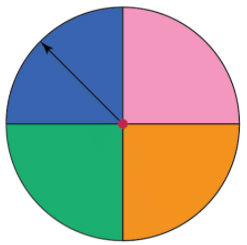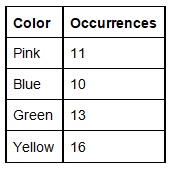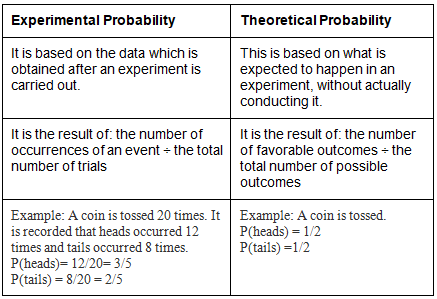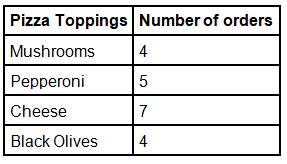# Probability: An Experimental Approach Notes | Study Mathematics (Maths) Class 9 - Class 9

## Class 9: Probability: An Experimental Approach Notes | Study Mathematics (Maths) Class 9 - Class 9

The document Probability: An Experimental Approach Notes | Study Mathematics (Maths) Class 9 - Class 9 is a part of the Class 9 Course Mathematics (Maths) Class 9.
All you need of Class 9 at this link: Class 9

Experimental Probability

The chance or occurrence of a particular event is termed as its probability. The value of a probability lies between 0 and 1 which means if it is an impossible event, the probability is 0 and if it is a certain event, the probability is 1. The probability that is determined on the basis of the results of an experiment is known as experimental probability. This is also known as empirical probability.

What is Experimental Probability?

Experimental probability is a probability which is determined on the basis of a series of experiments. A random experiment is done and is repeated many times to determine their likelihood and each repetition is known as a trial. The experiment is conducted to find the chance of an event to occur or not to occur. It can be tossing a coin, rolling a die, or rotating a spinner. In mathematical terms, the probability of an event is equal to the number of times an event occurred ÷ the total number of trials. For instance, you flip a coin 30 times and record whether you get a head or a tail. The experimental probability of obtaining a head is calculated as a fraction of the number of recorded heads and the total number of tosses. P(head) = Number of heads recorded ÷ 30 tosses.

Experimental Probability Formula

The experimental probability of an event is based on the number of times the event has occurred during the experiment and the total number of times the experiment was conducted. Each possible outcome is uncertain and the set of all the possible outcomes is called the sample space. The formula to calculate experimental probability is: P(E) = Number of times an event occurs/Total number of times the experiment is conducted.

Consider an experiment of rotating a spinner 50 times. The table given below shows the results of the experiment conducted. Let us find the experimental probability of spinning the color - blue.Experimental ProbabilityThe experimental probability of spinning the color blue = 10/50 = 1/5 = 0.2 = 20%

Experimental Probability vs Theoretical Probability

Experimental results are unpredictable and may not necessarily match the theoretical results. The results of experimental probability are close to theoretical only if the number of trials are more in number. Let us see the difference between experimental probability and theoretical probability.Experimental Probability Examples

Here are a few examples from real life scenarios.

1. The number of cookies made by Patrick per day in this week is given as 4, 7, 6, 9, 5, 9, 5.
Based on this data, what is the reasonable estimate of the probability that Patrick makes less than 6 cookies the next day?
P(< 6 cookies) = 3/7 = 0.428 = 42%
2. Find the reasonable estimate of the probability that while ordering a pizza, the next order will not be of a pepperoni topping.Based on this data, the reasonable estimate of the probability that the next type of toppings that would get ordered is not a pepperoni will be 15/20 = 3/4 = 75%

The document Probability: An Experimental Approach Notes | Study Mathematics (Maths) Class 9 - Class 9 is a part of the Class 9 Course Mathematics (Maths) Class 9.
All you need of Class 9 at this link: Class 9Use Code STAYHOME200 and get INR 200 additional OFF

## Mathematics (Maths) Class 9

84 videos|352 docs|109 tests

Track your progress, build streaks, highlight & save important lessons and more!

,

,

,

,

,

,

,

,

,

,

,

,

,

,

,

,

,

,

,

,

,

;